Past Year Questions: Highway Geometric Design & Planning - 1

# Past Year Questions: Highway Geometric Design & Planning - 1 - Notes | Study Transportation Engineering - Civil Engineering (CE)

 1 Crore+ students have signed up on EduRev. Have you?

Question 1. A parabolic vertical curve is being designed to join a road of grade +5% with a road of grade -3%. The length of die vertical curve is 400 m measured along the horizontal, The vertical point of curvature (VPC) is located an the road of grade +5%. The difference in height between VPC and vertical point of intersection (VPI) (in m, round off to the nearest integer) is _____.    [2019 : 2 Marks, Set-I]
Solution: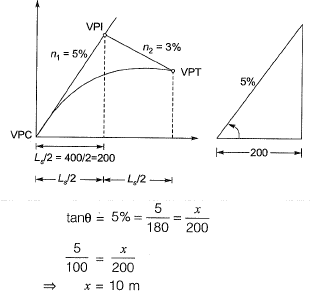Question 2.  The coefficient of average rolling friction of a road is fr, and its grade is +G%. If the grade of this road is doubled, what will be the percentage change in the braking distance (for the design vehicle to come to a stop) measured along the horizontal (assume all other parameters are kept unchanged)?    [2019 : 1 Mark, Set-I]
(a)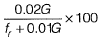(b)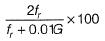(c)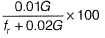(d)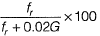Solution:
Percentage change in Braking Distance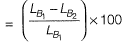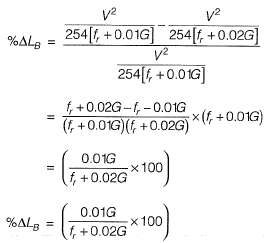Question 3. A car follows a slow moving truck (travelling at a speed of 10 m/s) on a two-lane two-way highway. The car reduces its speed to 10 m/s and follows the truck maintaining a distance of 16 m from the truck. On finding a clear gap in the opposing traffic stream, the car accelerates at an average rate of 4 m/s2, overtakes the truck and returns to its original lane. When it returns to its original lane, the distance between the car and the truck is 16 m. The total distance covered by the car during this period (from the time it leaves its lane and subsequently returns to its lane after overtaking) is    [2018 : 2 Marks, Set-II]
(a) 64 m
(b) 72 m
(c) 128 m
(d) 144 m
Solution:
Overtaking time,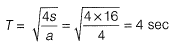S = Space heady way = 16 m
a = Acceleration = 4 m/s2
Distance travelled by vehicle = S2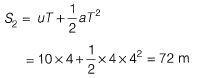Question 4. A 7.5 m wide two-lane road on a plain terrain is to be laid along a horizontal curve of radius 510 m. For a design speed of 100 kmph, superelevation is provided as per IRC: 73-1980. Consider acceleration due to gravity as 9.81 m/s2. The level difference between the inner and outer edges of the road (in m, up to three decimal places) is _______ .     [2018 : 2 Marks, Set-II]
Solution: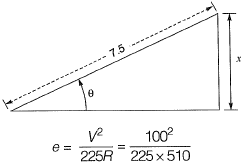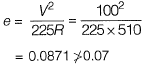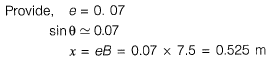Question 5. A priority intersection has a single-lane one-way traffic road crossing an undivided two-lane two- way traffic road. The traffic stream speed on the single-lane load is 20 kmph and the speed on the two-lane road is 50 kmph. The perception- reaction time is 2.5 s, coefficient of longitudinal friction is 0.38 and acceleration due to gravity is 9.81 m/s2. A clear sight triangle has to be ensured at this intersection. The minimum lengths of the sides of the sight triangle along the two-lane road and the single-lane road, respectively will be   [2018 : 2 Marks, Set-I]
(a) 50 m and 20 m
(b) 61m and 18 m
(c) 111m and 15 m
(d) 122 m and 36 m
(c)

Question 6. While aligning a hill road with a ruling gradient of 6%, a horizontal curve of radius 50 m is encountered. The grade compensation (in percentage, up to two decimal places) to be provided for this case would be __________ .    [2017 : 1 Mark, Set-II]
Solution: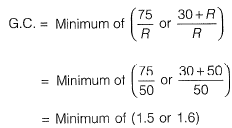Question 7.  The radius of a horizontal circular curve on a highway is 120 m. The design speed is 60 km/ hour, and the design coefficient of lateral friction between the tyre and the road surface is 0.15. The estimated value of superelevation required (if full lateral friction is assumed to develop), and the value of coefficient of friction needed (if no superelevation is provided) will, respectively, be    [2017 : 2 Marks, Set-I]
(a)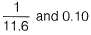(b)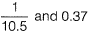(c)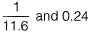(d)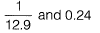(c)
Given, R = 120 m, V = 60 kmph and f = 0.15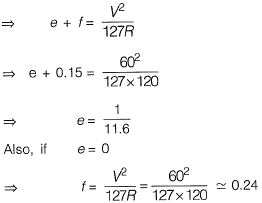Question 8.  A super-elevation e is provided on a circular horizontal curve such that a vehicle can be stopped on the curve without sliding. Assuming a design speed v and maximum coefficient of side friction fmax, which one of the following criteria should be satisfied?    [2017 : 1 Mark, Set-I]
(a) e ≤ fmax
(b) e > fmax
(c) no limit on e can be set
(d)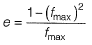(a)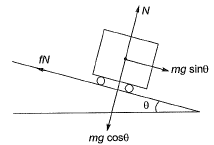Sliding of stopped vehicle occurs if,
mg sinθ > fn
mg sinθ > f(mg cosθ)

tanθ > f
e> f
Hence, to prevent sliding e ≤ fmax

Question 9. A motorist traveling at 100 km/h on a highway needs to take the next exit, which has a speed limit of 50 km/h. The section of the roadway before the ramp entry has a downgrade of 3% and coefficient of friction (f) is 0.35. In order to enter the ramp at the maximum allowable speed limit, the braking distance (expressed in m) from the exit ramp is_______.    [2016 : 2 Marks, Set-II]
Solution: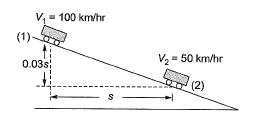Total energy lost between point (1) and (2)
= Work done by frictional force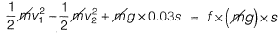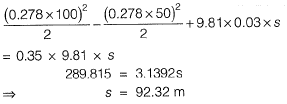Question 10.  A two lane, one-way road with radius of 50 m is predominantly carrying lorries with wheelbase of 5 m. The speed of lorries is restricted to be between 60 kmph and 80 kmph. The mechanical widening and psychological widening required at 60 kmph are designated as Wme 60 and Wps 60, respectively. The mechanical widening and psychological widening required at 80 kmph are designated as Wme 80 and Wps 80, respectively. The correct values of Wme 60, Wps 60, Wme 80, Wps 80, respectively are    [2016 : 2 Marks, Set-I]
(a) 0.89 m, 0.50 m, 1.19 m, and 0.50 m
(b) 0.50 m, 0.89 m, 0.50 m, and 1.19 m
(c) 0.50 m, 1.19 m, 0.50 m, and 0.89 m
(d) 1.19 m, 0.50 m, 0.89 m, and 0.50 m
Solution:
Method-I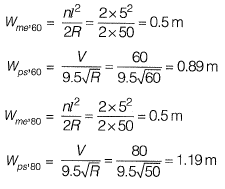Method-II
As mechanical widening is independent of speed of the vehicle,
Wmc’60= Wme,80
Hence, options (a) and (b) are eliminated.
Now, as psychological widening is directly proportional to the speed of the vehicle.
Wps,60 < Wps,80 Hence, option (b) is the correct answer.

Question. 11  A superspeedway in New Delhi has among the highest super-elevation rates of any track on the Indian Grand Prix circuit. The track requires drivers to negotiate turns with a radius of 335 m and 33° banking. Given this information, the coefficient of side friction required in order to allow a vehicle to travel at 320 km/h along the curve is    [2015 : 1 Mark, Set-II]
(a) 1.761
(b) 0.176
(c) 0.253
(d) 2.530

Solution: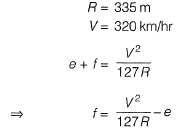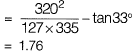Note: While deriving the above equation, we consider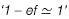but this is not valid in this case because banking provided is very high (tan 33° = 0.65). So formula used should be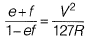which will result in f = 0.685.

The document Past Year Questions: Highway Geometric Design & Planning - 1 - Notes | Study Transportation Engineering - Civil Engineering (CE) is a part of the Civil Engineering (CE) Course Transportation Engineering.
All you need of Civil Engineering (CE) at this link: Civil Engineering (CE)

## Transportation Engineering

22 videos|68 docs|39 tests
 Use Code STAYHOME200 and get INR 200 additional OFF

## Transportation Engineering

22 videos|68 docs|39 tests

Track your progress, build streaks, highlight & save important lessons and more!

,

,

,

,

,

,

,

,

,

,

,

,

,

,

,

,

,

,

,

,

,

;